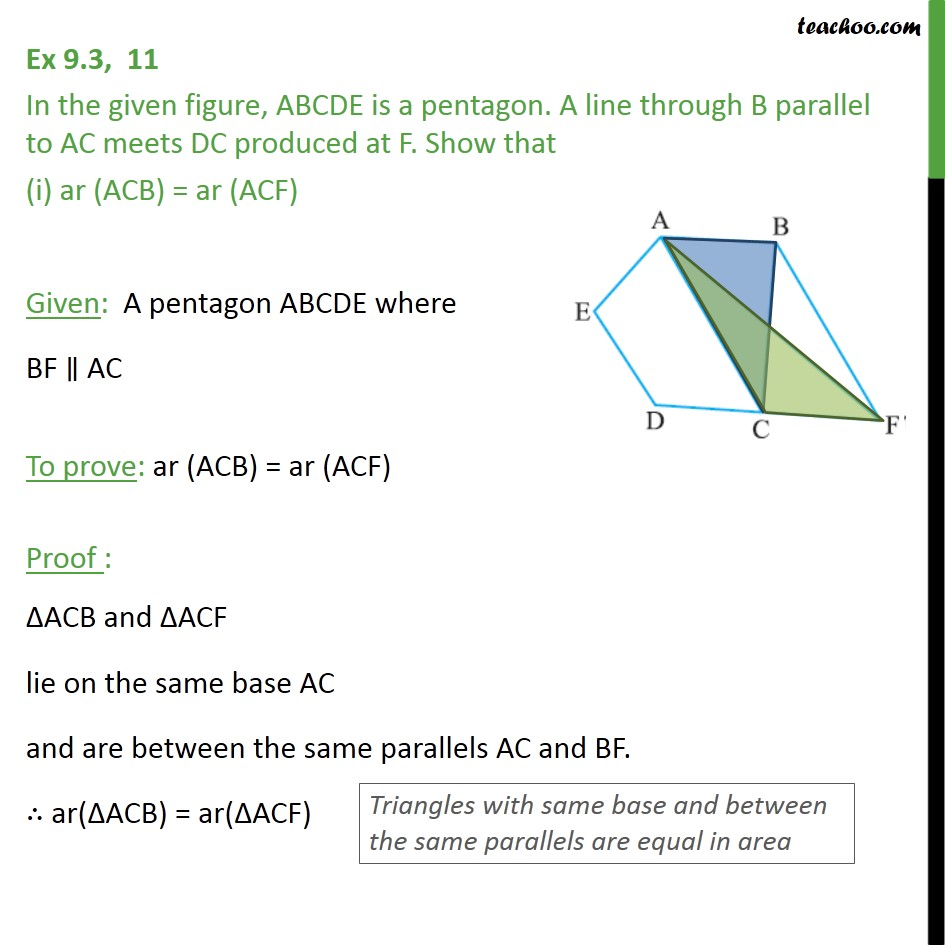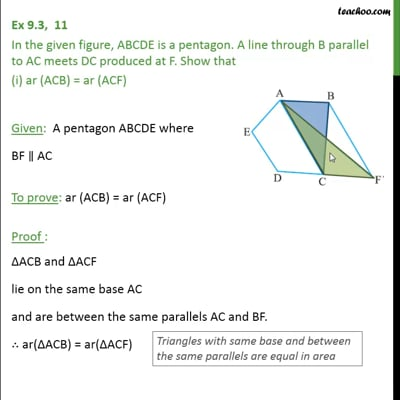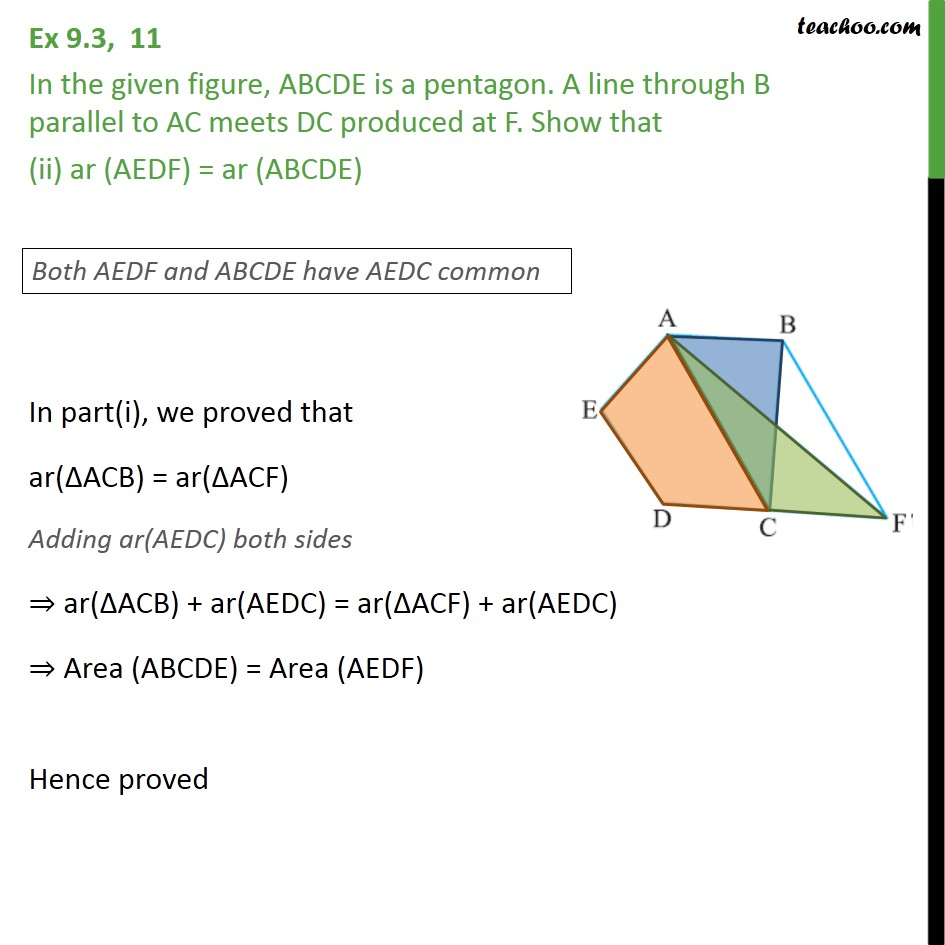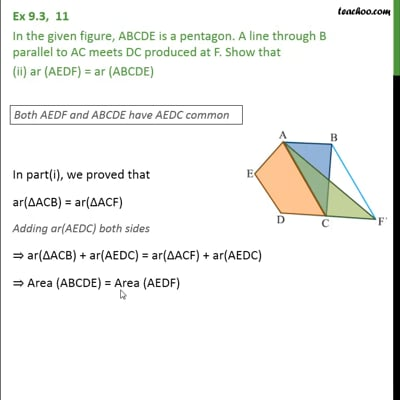Area of Triangles

Areas of Parallelograms and Triangles
Serial order wiseThis video is only available for Teachoo black usersThis video is only available for Teachoo black users

Learn in your speed, with individual attention - Teachoo Maths 1-on-1 Class

### Transcript

Question 11 In the given figure, ABCDE is a pentagon. A line through B parallel to AC meets DC produced at F. Show that (i) ar (ACB) = ar (ACF) Given: A pentagon ABCDE where BF AC To prove: ar (ACB) = ar (ACF) Proof : ACB and ACF lie on the same base AC and are between the same parallels AC and BF. ar( ACB) = ar( ACF) Question 11 In the given figure, ABCDE is a pentagon. A line through B parallel to AC meets DC produced at F. Show that (ii) ar (AEDF) = ar (ABCDE) In part(i), we proved that ar( ACB) = ar( ACF) Adding ar(AEDC) both sides ar( ACB) + ar(AEDC) = ar( ACF) + ar(AEDC) Area (ABCDE) = Area (AEDF) Hence proved